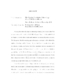## The Relative Lie Algebra Cohomology of the Weil Representation2015
##### Authors
Ralston, Jacob
We study the relative Lie algebra cohomology of $\mathfrak{so}(p,q)$ with values in the Weil representation $\varpi$ of the dual pair $\mathrm{Sp}(2k, \R) \times \OO(p,q)$. Using the Fock model defined in Chapter \ref{Introchapter}, we filter this complex and construct the associated spectral sequence. We then prove that the resulting spectral sequence converges to the relative Lie algebra cohomology and has $E_0$ term, the associated graded complex, isomorphic to a Koszul complex, see Section \ref{defofkoszulsection}. It is immediate that the construction of the spectral sequence of Chapter \ref{spectralchapter} can be applied to any reductive subalgebra $\mathfrak{g} \subset \mathfrak{sp}(2k(p+q), \R)$. By the Weil representation of $\OO(p,q)$, we mean the twist of the Weil representation of the two-fold cover $\widetilde{\OO(p,q)}$ by a suitable character. We do this to make the center of $\widetilde{\OO(p,q)}$ act trivially. Otherwise, all relative Lie algebra cohomology groups would vanish, see Proposition \ref{genuineprop}. In case the symplectic group is large relative to the orthogonal group ($k \geq pq$), the $E_0$ term is isomorphic to a Koszul complex defined by a regular sequence, see \ref{defofkoszulsection}. Thus, the cohomology vanishes except in top degree. This result is obtained without calculating the space of cochains and hence without using any representation theory. On the other hand, in case $k < p$, we know the Koszul complex is not that of a regular sequence from the existence of the class $\varphi_{kq}$ of Kudla and Millson, see \cite{KM2}, a nonzero element of the relative Lie algebra cohomology of degree $kq$. For the case of $\SO_0(p,1)$ we compute the cohomology groups in these remaining cases, namely $k < p$. We do this by first computing a basis for the relative Lie algebra cochains and then splitting the complex into a sum of two complexes, each of whose $E_0$ term is then isomorphic to a Koszul complex defined by a regular sequence. This thesis is adapted from the paper, \cite{BMR}, this author wrote with his advisor John Millson and Nicolas Bergeron of the University of Paris.